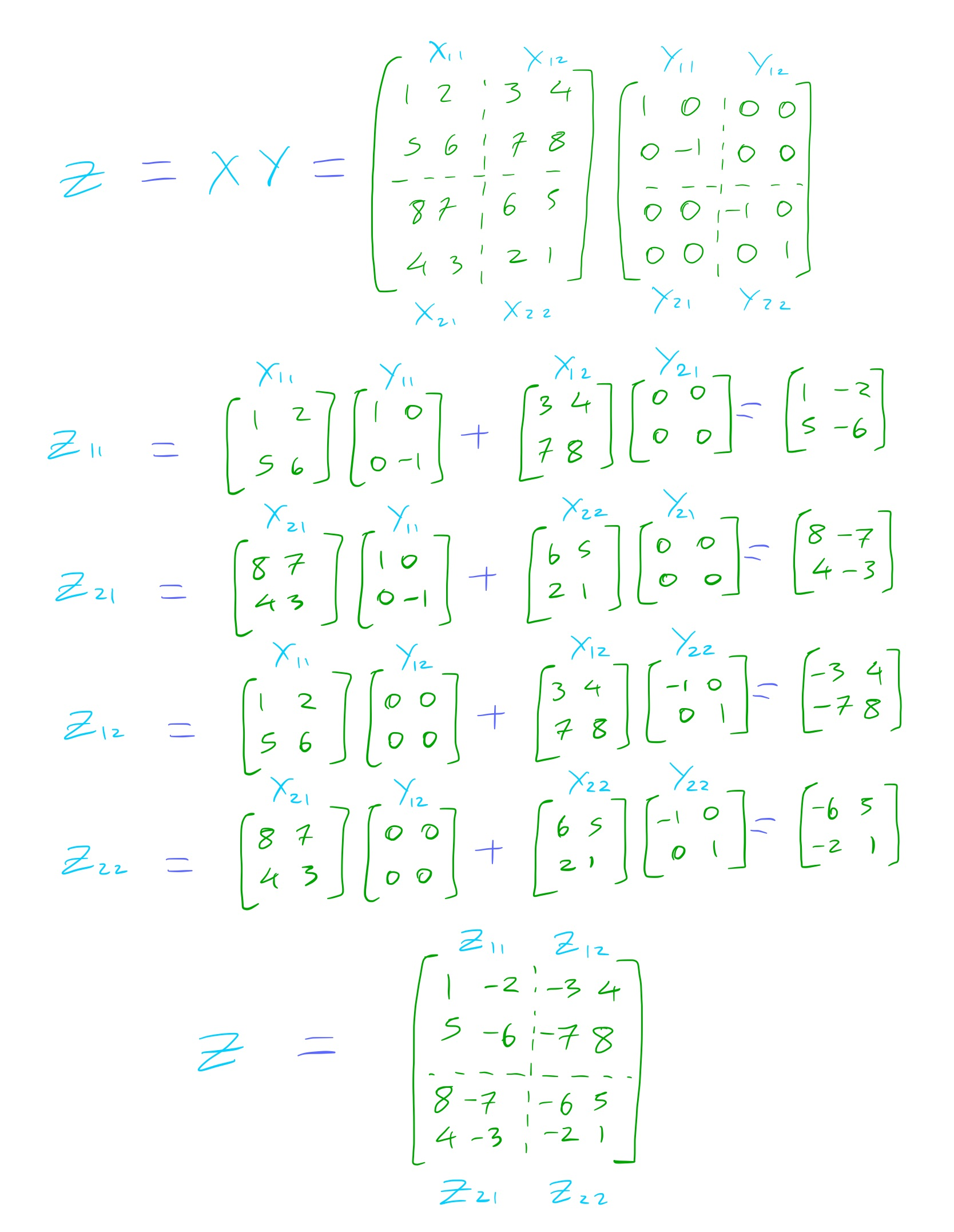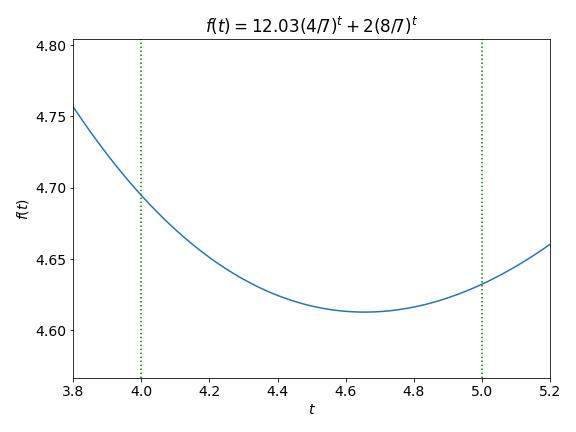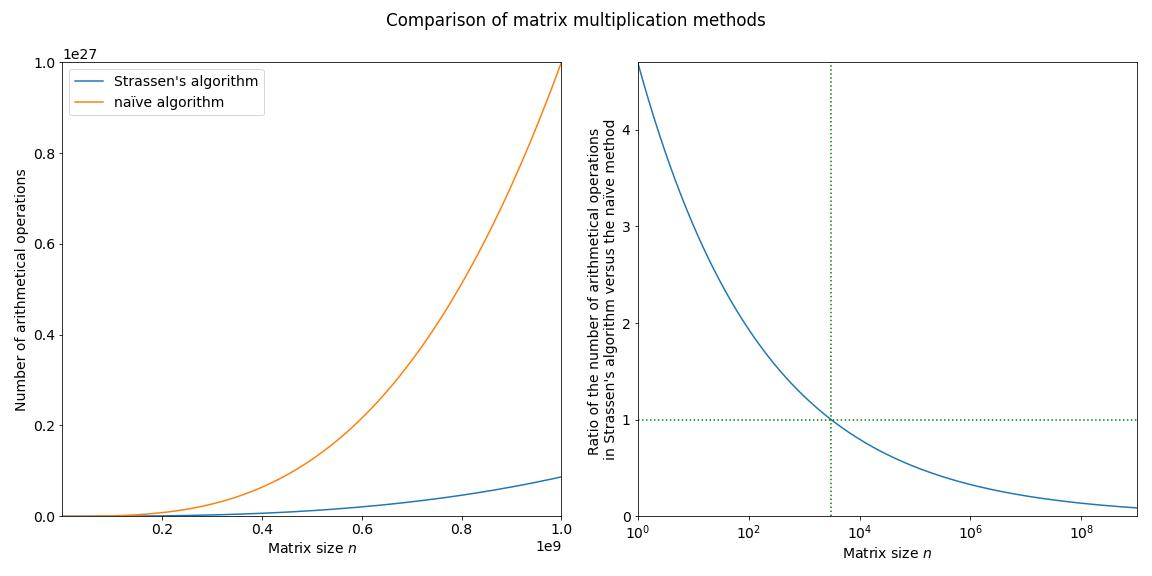## Introduction

This is the first of a planned series of blogs covering background topics for DeepMind’s AlphaTensor paper. In this post we will cover Strassen’s algorithm for matrix multiplication.

Matrices matter to an extent unimaginable in in 1969 when the paper proposing this method was published and methods to multiply them fast are crucial. Most notably they are key to deep learning training and inference so it is very exciting that by using deep learning methods, new algorithms for fast matrix multiplication have been discovered.

## The complexity of matrix multiplication

If you are reading this article, then you almost certainly know the naïve method for multiplying two matrices. Restricting ourselves to square $n \times n$ matrices for simplicity, this can written as

$Z = XY \\Z_{ij} = \sum_{t=1}^n X_{it}Y_{tj}$

To compute each element $Z_{ij}$ you need $n$ multiplications and $n-1$ additions and there are $n^2$ such elements leading to a total of $n^3$ multiplications and $n^2(n-1)$ additions so $n^2(2n - 1) = O(n^3)$ arithmetical operations in total.

Laid out like this it seems almost obvious that matrix multiplication requires $O(n^3)$ arithmetical operations. It turns out that it is a strictly simpler problem.

## A recursive algorithm for matrix multiplication

Strassen’s algorithm relies on the fact that the matrix multiplication formula is valid when $X_{ij}$, $Y_{ij}$ and $Z_{ij}$ are themselves matrices rather than numbers. This allows you to divide the matrix into blocks and work with them instead, as you can see in the example belowHowever simply recasting the naïve method to a recursive form is not enough. To gain an improvement the computations need to happen in a different way and you can find out how by reading the paper yourself.

### Exercise 1: The algorithm

Read the paper and optionally implement the algorithm.

The paper is less than 3 pages in length and quite easy to follow. Focus on the first two pages which cover multiplication (since the method can also be extended to finding inverses and determinants). Don’t worry if you don’t understand all the details. In the subsequent exercises we will derive some of the results in greater detail.

If implementing, I recommend not using any libraries like NumPy or similar libraries in other languages but representing the matrices as arrays and defining your own matrix multiplication and addition functions.

def matmul(X, Y):
(size, _), _ = shapeX, shapeY = list(map(get_shape, [X, Y]))
# Restrict to square
assert (set(shapeX + shapeY)) == {size}
Z = zeros_square(size)
for i in range(size):
for j in range(size):
for t in range(size):
Z[i][j] += (X[i][t] * Y[t][j])
return Z

(size, _), _ = shapeX, shapeY = list(map(get_shape, [X, Y]))
# Restrict to square
assert (set(shapeX + shapeY)) == {size}
Z = zeros_square(size)
for i in range(size):
for j in range(size):
Z[i][j] = X[i][j] + Y[i][j]

return Z

def matsub(X, Y):
(size, _), _ = shapeX, shapeY = list(map(get_shape, [X, Y]))
# Restrict to square
assert (set(shapeX + shapeY)) == {size}
Z = zeros_square(size)
for i in range(size):
for j in range(size):
Z[i][j] = X[i][j] - Y[i][j]

return Z

def get_shape(X):
return (len(X), len(X))

def zeros_square(size):
return [[0 for _ in range(size)] for _ in range(size)]

def split(X):
size = len(X)
halfsize = size // 2
result = [[zeros_square(halfsize) for _ in range(2)] for
_ in range(2)]

assert (halfsize *2) == size
for i in range(size):
for j in range(size):
result[i//halfsize][j//halfsize][i%halfsize][j%halfsize] = X[i][j]

return result

def merge(splits):
halfsize = len(splits)
size = halfsize * 2
result = zeros_square(size)

for i in range(size):
for j in range(size):
result[i][j] = splits[i//halfsize][j//halfsize][i%halfsize][j%halfsize]

return result

def strassen_matmul(X, Y, return_params=False):
(size, _), _ = shapeX, shapeY = list(map(get_shape, [X, Y]))
# Restrict to square
assert (set(shapeX + shapeY)) == {size}

# It might be more optimal in somes cases to round up to the nearest power of 2
# and pad the matrix accordingly but we will keep things simple here
# for demo purposes and express the size in form m*2^k

k = 0
m = size
while (m % 2) == 0:
m = m // 2
k += 1

if k == 0:
return matmul(X, Y)

[[X11, X12], [X21, X22]] = split(X)
[[Y11, Y12], [Y21, Y22]] = split(Y)

III = strassen_matmul(X11, matsub(Y12, Y22))
IV = strassen_matmul(X22, matsub(Y21, Y11))
VI = strassen_matmul(matsub(X21, X11), matadd(Y11, Y12))
VII = strassen_matmul(matsub(X12, X22), matadd(Y21, Y22))

Z = merge([[Z11, Z12], [Z21, Z22]])

return (Z, k, m) if return_params else Z

# Example
import numpy as np # using np just for testing
s = 80 # = 2^4 * 5
A = np.random.randint(0, 100, (s, s))
B = np.random.randint(0, 100, (s, s))
Z = A @ B
Al, Bl = map(np.ndarray.tolist, [A, B])
assert (matmul(Al, Bl) == Z).all()
assert (merge(split(A)) == A).all()
assert (matadd(Al, Bl) == (A + B)).all()
assert (matsub(Al, Bl) == (A - B)).all()
ZZ, kk, mm = strassen_matmul(Al, Bl, return_params=True)
assert (ZZ == Z).all()
print(kk, mm) # => 4 5


### Exercise 2: Number of additions and multiplications

Let $A(k)$ denote the number of additions and $M(k)$ the number of multiplications needed to multiply 2 $m 2^k$ square matrices. We know that $M(0) = m^3$ and $A(0) = m^2(m - 1)$.

Show the following from Fact 1 in the paper

$M(k) = 7^k m^3$ $A(k) = (5 + m)m^2 7^k - 6(m2^k)^2$

There is one matrix multiplication in steps $I-IV$ so 7 in total, involving the sub-matrices of size $m 2^{k-1}$, each of which contributes $M(k-1)$ multiplication operations

$M(k) = 7M(k-1) = 7^2M(k-2)= \cdots = 7^kM(0) = 7^k m^3$

There are 18 matrix additions, 10 in $I-IV$ and $8$ in the subsequent steps, involving the sub-matrices of size $m 2^{k-1}$ leading to $18(m 2^{k-1})^2$ addition operations. But there are also the additions involved in the 7 matrix multiplications in $I-IV$, each of which contributes contributes $A(k-1)$ additions

$A(k) = 18 m^2 4^{k-1} + 7A(k-1) \\= 18 m^2 4^{k-1} + 7\cdot18 m^2 4^{k-2} + 7^2A(k-2) \\= 18 m^2 4^{k-1} + 7\cdot18 m^2 4^{k-2} + 7^2\cdot18 m^2 4^{k-3} + 7^3A(k-3) \\= \cdots \\ = (18m^24^{k-1})\sum_{t=0}^{k-1}(7/4)^t + 7^kA(0) \\ = (18m^24^{k-1})\frac{(7/4)^k - 1}{7/4 - 1} + 7^km^2(m-1) \\ = (18m^24^{k-1})\frac{7^k/4^{k-1} - 4}{3} + 7^km^2(m-1) \\ = 6m^2\left(7^k - 4^k\right) + 7^km^2(m-1) \\ = (5 + m)m^2 7^k - 6(m 2^k)^2$

## Complexity proof

I think the the notation $[x]$ here refers to the floor function or the integral part of the number which for a positive number is equivalent to floor. In any case from the way Fact 2 is used in the rest of the paper it would appear that

$x - 1 \leq [x] \leq x$

### Exercise 3: Proof of fact 2, part 1

Show that

$(5 + 2m)m^27^k - 6(m2^k)^2 < 2n^3(7/8)^k + 12.03n^2(7/4)^k$

We know that

$m = \left[n 2^{-k}\right] + 1 \\ \implies \left(n 2^{-k} - 1\right) + 1 \leq m \leq n 2^{-k} + 1 \\ \implies n 2^{-k} \leq m \leq n 2^{-k} + 1$

Hence

$(5 + 2m)m^27^k - 6(m2^k)^2 \\ \leq \left(5 + 2\left(n 2^{-k} + 1\right)\right)\left(n 2^{-k} + 1\right)^27^k - 6\left(\left(n 2^{-k} + 1\right)2^k\right)^2 \\ \lt \left(5 + 2\left(n 2^{-k} + 1\right)\right)\left(n 2^{-k} + 1\right)^27^k$

since $6\left(\left(n 2^{-k} + 1\right)2^k\right)^2 > 0$.

$=7^{k} \left(7 + 16 n2^{- k} + 11 n^{2}2^{- 2 k} + 2 n^{3}2^{- 3 k}\right) \\=7^{k}\left(7 + 16 n2^{- k} + 11 \left(n 2^{-k}\right)^2 + 2 \left(n 2^{-k}\right)^3\right)$

and as we have that $k \leq \log_2 n - 4 \implies 16\leq n 2^{-k}$

$\leq 7^{k}\left((7/256)\left(n2^{- k}\right)^2 + \left(n2^{- k}\right)^2 + 11 \left(n 2^{-k}\right)^2 + 2 \left(n 2^{-k}\right)^3\right) \\= 7^{k}\left((11 + 263 / 256) \left(n 2^{-k}\right)^2 + 2 \left(n 2^{-k}\right)^3\right) \\ < 12.03 n^2(7/4)^k + 2 n^3(7/8)^k$

### Exercise 4: Proof of fact 2, part 2

Show that

$2n^3(7/8)^k + 12.03n^2(7/4)^k \leq \left(2(8/7)^{\log_2 n - k} + 12.03(4/7)^{\log_2 n - k}\right)n^{\log_2 7}$

Since $n = 2^{\log_2 n}$

$2n^3(7/8)^k + 12.03n^2(7/4)^k \\=\left(2\left(8^{\log_2 n}/8^k\right)(7^k/7^{\log_2 n}) + 12.03\left(4^{\log_2 n}/4^k\right) (7^k/7^{\log_2 n})\right)7^{\log_2 n}$

and since $7^{\log_2 n} = \left(2^{\log_2 7}\right)^{\log_2 n} = \left(2^{\log_2 n}\right)^{\log_2 7} = n^{\log_2 7}$

$=\left(2(8/7)^{\log_2 n - k} + 12.03(4/7)^{\log_2 n - k}\right)n^{\log_2 7}$

### Exercise 5: Proof of fact 2, final part

Here we will finally prove that the number of operations needed is strictly less than $O(n^3)$.

Show that

$\left(2(8/7)^{\log_2 n - k} + 12.03(4/7)^{\log_2 n - k}\right)n^{\log_2 7} \leq 4.7 \cdot n^{\log_2 7}$

• If a function $f$ is convex then for any two values $x, y$ in the domain of $f$, and $0 \leq \theta \leq 1$
$f(\theta x + (1 - \theta)y) \leq \theta\cdot f(x) + (1 - \theta) \cdot f(y)$
• Assuming we are only dealing with real numbers, all the following are convex functions
• $e^{ax}$ for any $a$ from which it follows that for $b>0$, $b^x$ is convex since it can be written in the form $e^{\mathrm{ln}(b)x}$
• A non-negative multiple of a convex function
• The sum of two convex functions

Let $t = \log_2 n - k$. We know that

$\left(\log n - 4\right) - 1 \leq k \leq \log n - 4 \\ \implies \log n - 5 \leq k \leq \log n - 4 \\ \implies 4 \leq \log n - k \leq 5 \\ \implies 4 \leq t \leq 5$

So the maximum value of the left hand side is given as

$\left(2(8/7)^{\log_2 n - k} + 12.03(4/7)^{\log_2 n - k}\right)n^{\log_2 7} \\ = \left(2(8/7)^t + 12.03(4/7)^t\right)n^{\log_2 7} \\ \leq \max_{4 \leq t \leq 5}\left(2(8/7)^t + 12.03(4/7)^t\right)n^{\log_2 7}$

Let $f(t) = 2(8/7)^t + 12.03(4/7)^t$. We wish to find the maximum of this function within the interval $4 \leq t \leq 5$. Note that both $2(8/7)^t$ and $12.03(4/7)^t$ are convex functions since they are positive multiples of functions of the form $b^t$ where $b > 0$. Since $f(t)$ is the sum of two convex functions, it is also convex.We can see that $f(4) \approx 4.7 \gt f(5) \approx 4.6$. From the plot it looks like $f(4)$ is maximum value of $f(t)$ in this interval and we can confirm this mathematically.

Any value $t$ in the interval can be expressed as

$t(\theta) = 4\theta + 5(1 - \theta) \\ 0 \leq \theta \leq 1$

Then we find that

$\forall t \in [4, 5] \\ f(t) = f\left(4\theta + 5(1 - \theta) \right) \\ \leq \theta \cdot f(4) + (1 - \theta)\cdot f(5)$

since $f$ is convex

$\leq \theta \cdot f(4) + (1 - \theta)\cdot f(4) \\ \leq f(4)$

Therefore

$\left(2(8/7)^{\log_2 n - k} + 12.03(4/7)^{\log_2 n - k}\right)n^{\log_2 7} \\ \leq 4.7\cdot n^{\log_2 7}$

## Conclusion

Compared to the original $O(n^3)$, less than $4.7\cdot n^{\log_2 7} \approx O(n^{2.8})$ arithmetical operations does not seem a terribly impressive result. Even so, it leads to non-negligible improvements for sufficiently large matrices.But what is important is that it raises the question of whether there aren’t even simpler algorithms. However the paper does not explain how this method comes about nor does it give any hints about how one might go about finding more optimal algorithms. AlphaTensor uses deep reinforcement learning to look for solutions.